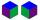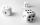# Cube 9

What was the original edge length of the cube if after cutting 39 small cubes with an edge length 2 dm left 200 dm3?

Result

x =  8 dm

#### Solution:Leave us a comment of example and its solution (i.e. if it is still somewhat unclear...):

Showing 0 comments:Be the first to comment!#### To solve this example are needed these knowledge from mathematics:

Tip: Our volume units converter will help you with converion of volume units.

## Next similar examples:

1. Cube containersTwo containers shaped of cube with edges of 0.7 m and 0.9 m replace a single cube so that it has the same volume as the original two together. What is the length of the edges of the new cube?
2. Cube cornersFrom cube of edge 14 cm cut off all vertices so that each cutting plane intersects the edges 1 cm from the nearest vertice. How many edges will have this body?
3. Two boxes-cubesTwo boxes cube with edges a=38 cm and b = 81 cm is to be replaced by one cube-shaped box (same overall volume). How long will be its edge?
4. Three diceWhen you throw three dice was the sum total of the dice 10. The yellow dice fell one eye more than on the red and brown fell 3 eyes less than red. How many eyes fell on every dice?
5. The volume 2The volume of a cube is 27 cubic meters. Find the height of the cube.
6. Theorem proveWe want to prove the sentense: If the natural number n is divisible by six, then n is divisible by three. From what assumption we started?
7. Functions f,gFind g(1) if g(x) = 3x - x2 Find f(5) if f(x) = x + 1/2
8. BottlesThe must is sold in 5-liter and 2-liter bottles. Mr Kucera bought a total of 216 liters in 60 bottles. How many liters did Mr. Kucera buy in five-liter bottles?
9. One halfOne half of ? is: ?
10. 6 termsFind the first six terms of the sequence. a1 = 7, an = an-1 + 6
11. Factory and divisionsThe factory consists of three auxiliary divisions total 2,406 employees. The second division has 76 employees less than 1st division and 3rd division has 212 employees more than the 2nd. How many employees has each division?
12. Two equationsSolve equations (use adding and subtracting of linear equations): -4x+11y=5 6x-11y=-5
13. PowersExpress the expression ? as the n-th power of the base 10.
14. Volleyball8 girls wants to play volleyball against boys. On the field at one time can be six players per team. How many initial teams of this girls may trainer to choose?
15. Linear systemSolve a set of two equations of two unknowns: 1.5x+1.2y=0.6 0.8x-0.2y=2
16. Nineteenth memberFind the nineteenth member of the arithmetic sequence: a1=33 d=5 find a19
17. TreesA certain species of tree grows an average of 0.5 cm per week. Write an equation for the sequence that represents the weekly height of this tree in centimeters if the measurements begin when the tree is 200 centimeters tall.